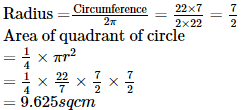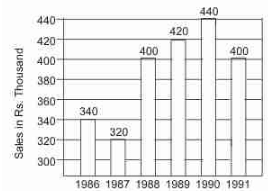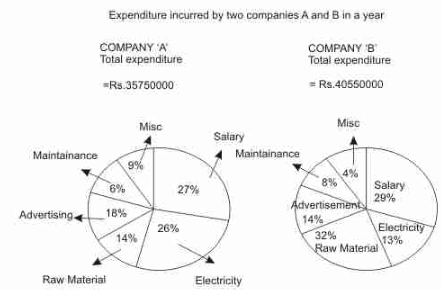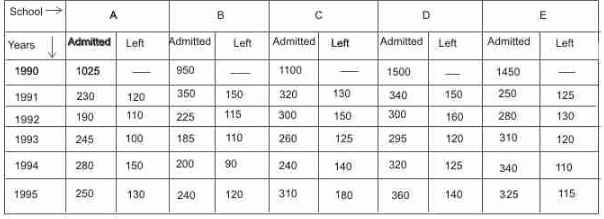# NABARD Manager Quant Aptitude Mock Test 2

## 20 Questions MCQ Test NABARD Manager - Mock Tests & Previous Year Papers | NABARD Manager Quant Aptitude Mock Test 2

Description
Attempt NABARD Manager Quant Aptitude Mock Test 2 | 20 questions in 12 minutes | Mock test for Banking Exams preparation | Free important questions MCQ to study NABARD Manager - Mock Tests & Previous Year Papers for Banking Exams Exam | Download free PDF with solutions
QUESTION: 1

### The age of father 10 years ago was thrice the age of his son. Ten years hence, father's age will be twice that of his son. The ratio of their present ages is:

Solution: Let the ages of father and son 10 years ago be 3x and x years respectively.
Then, (3x + 10) + 10 = 2[(x + 10) + 10]
⇒ 3x + 20 = 2x + 40
⇒ x = 20
∴ Required ratio = (3x + 10) : (x + 10) = 70 : 30 = 7 : 3
QUESTION: 2

### The area of the quadrant of a circle whose circumference is 22 cm is

Solution:QUESTION: 3

### A house worth Rs 150000 is sold by X at 5% profit to Y. Y sells the house back to X at a 2% loss. Then, in the entire transaction

Solution:

CP = Rs 150000
Overall gain percentage
= 5 - 2 - 10 100 = 2.9%
⇒ 150000 x 29/ 1000 = Rs 4350 gain

QUESTION: 4
The mean of 50 observations was 36. It was found later that an observation 48 was wringly taken as 23. The corrected new mean is:
Solution:
QUESTION: 5

Which fraction comes next in the sequence 1/ 2 , 3 /4 , 5/ 8 , 7/ 16 , ?

Solution:

Clearly, the numerators of the fractions in the given sequence form
the series 1,3,5,7 in which each term is obtained by adding 2 to the previous terms
The denominators of the fractions form the series
2, 4, 8, 16 i.e. 21, 22, 23, 24
So, the numerator of the next fraction will be (7 + 2) i.e. 9
and the denominator will be 25
Thus, the next term is 9 32

QUESTION: 6

A property tax increase was proposed by a municipal corporation and it was observed that 40% of the property owners favoured it while 80% of the nonowners of property favoured it. If 70% of voters are property owners, what is the probability that a voter selected at random would be the one favouring the increase?

Solution:

Favourable number of cases
= 40 x 70 /100 + 30 x 80/ 100
= 28 + 24 = 52
∴ Required probability
= 52 100 = 0.52

QUESTION: 7
A gets 20% more money than B. How much % money B gets less than A
Solution: If B gets 100, A gets 120 and % B gets less than $A=\frac{20}{120}�100=16\frac{2}{3}%$
QUESTION: 8

The ratio of the monthly income to the savings of a family is 9:2. If the monthly income of the family is Rs 2700, then its monthly expenditure will be

Solution:

Income/ Savings
= 9 2
⇒ 2700 /x = 9/ 2 ⇒ x = 600
Hence, Expenditure = 2700 - 600 = Rs 2100

QUESTION: 9
The simple interest on a sum of money is 1/4 of the principal. If number of years is equal to rate percent per annum, the interest rate is
Solution:
QUESTION: 10
Two cans A and B contain milk and water in the ratio 4:1 and 5:2 respectively. Find the ratio in which these mixtures are to be mixed to make a new mixture containing milk and water in the ratio 7:2.
Solution:
QUESTION: 11
Six labourers complete a piece of work in 20 days. If only four labourer are employed, then the time taken by them will be
Solution:
QUESTION: 12
The base radii of two cylinders are in the ratio 2 : 3 and their heights are in the ratio 5 : 3. The ratio of their volumes is
Solution:
QUESTION: 13

In each of these questions two equations numbered I & II are given. You have to solve both the equations and give answer

I. a2 + 5a + 6 = 0
II. b2 + 3b + 2 = 0

Solution:

I. a2 + 5a + 6 = 0
⇒ a2 + 2a + 3a + 6 = 0
⇒ a (a + 2) + 3 (a + 2) = 0
⇒ (a + 2) (a + 3) = 0
⇒ a = -2 or -3
II. b2 + 3b + 2 = 0
⇒ b2 + 2b + b + 2 = 0
⇒ b (b + 2) + 1 (b + 2) = 0
⇒ (b + 1) (b + 2) = 0
⇒ b = -1 or -2
a ≤ b

QUESTION: 14

In each of these questions two equations numbered I & II are given. You have to solve both the equations and give answer

I. 2a2 + 3a + 1 = 0
II. 12b2 + 7b + 1 = 0

Solution:

I. 2a2 + 3a + 1 = 0
⇒ 2a2 + 2a + a + 1 = 0
⇒ 2a (a + 1) + 1 (a + 1) = 0
⇒ (a + 1) (2a + 1) = 0
⇒ a = -1 or - 1212
II. 12b2 + 7b + 1 = 0
⇒ 12b2 + 3b + 4b + 1 = 0
⇒ 3b (4b + 1) + 1 (4b + 1) = 0
⇒ (3b + 1) (4b + 1) = 0
⇒ b = - 1/3 or - 1/4
a < b

QUESTION: 15By how much amount are the sales in 1989 more than that in 1987 ?

Solution:
QUESTION: 16The sales in 1987 are how many times to that in 1988 ?

Solution:

In 1989 sales = 420 thousand
1987 sales = 320 thousand
So sales in 1989 more than in 1987 = 420000 - 320000 = 100000

QUESTION: 17

Study the following graphs carefully to answer the questions given below it.What is the amount (in Rs.) spent by Company 'A' on Electricity ?

Solution:
QUESTION: 18

Study the following graphs carefully to answer the questions given below it.How much (in Rs.) have both companies together spent on raw material ?

Solution:
QUESTION: 19

Read the following information carefully and answer the questions based on it.
In 6 educational years, number of students taking admission and leaving from the 5 different school which are founded in 1990 are given belowWhat is the average number of students studying in all the five schools in 1992 ?

Solution:

Total no. of students studying in all schools in 1992
= (1025 + 230 + 190 + 950 + 350 + 225 + 1100 + 320 + 300 + 1500 + 340 + 300 + 1450 + 250 + 280) - (120 + 110 + 150 + 115 + 130 + 150 + 150 + 160 + 125 + 130)
= 8810 - 1340 = 7470
∴ Average = 7470/5 = 1494

QUESTION: 20

Read the following information carefully and answer the questions based on it.
In 6 educational years, number of students taking admission and leaving from the 5 different school which are founded in 1990 are given belowWhat was the number of students studying in School B in 1994 ?

Solution:

Number of students studying in school B in 1994
= 950 + (350 - 150) + (225 - 115) + (185 - 110) + (200 - 90)
= 950 + 200 + 110 + 75 + 110 = 1445Use Code STAYHOME200 and get INR 200 additional OFF Use Coupon Code